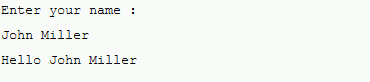## getline Function in c++

Well as we know cin is an object which is used to take input from the user but does not allow to take the input in multiple lines.To accept the multiple lines as input we use getline c++

It is a pre-defined function in C++ which is defined in a <string.h> header file getline function c++ is used to  accept a line or a string from the input stream until the delimiting character is encountered by compiler.

# Syntax of getline() C++ function:

this function can be represent in two way  :-

• The first way of declaring function is to pass three parameters.
1. istream& getline( istream& is, string& str, char delim );

the three parameter are  is, str, and delim.

Where :-

is: It is an object which is used from where to read the input stream.

str object: is used to stored a string.

delim: delimiting character.

Return value

getline function c++ returns the input stream object, which is passed as a parameter to the getline function.

• The second mwthod of declaring is to pass two parameters.

istream& getline( istream& is, string& str );

as you can in above syntax we have two parameters whicch are is and str.

this method does not have any delimiting character like first one.

is: It is an object which is used from where to read the input stream.

str object: is used to stored a string.

## Example of getline c++

In this example we will take the input from user without using getline() function in C++

```#include <iostream>
#include  <string.h>
using namespace std;
int main()
{
string name; // variable declaration
std::cout << "Enter your name :" << std::endl;
cin>>name;
cout<<"\nHello "<<name;
return 0;
}```

OUTPUTOUTPUT
As you see we give 'John Miller' as user input, but only 'John' was displayed in output.

## Let's resolve the above problem by using getline() C++ .

``` #include <iostream>
#include  <string.h>
using namespace std;
int main()
{
string name; // variable declaration.
std::cout << "Enter your name :" << std::endl;
getline(cin,name); // implementing a getline() function
cout<<"\nHello ""<<name;
return 0;
}

```

In the above example we can get the full name with the help of getline function c++.

OUTPUTOUTPUT getline function c++